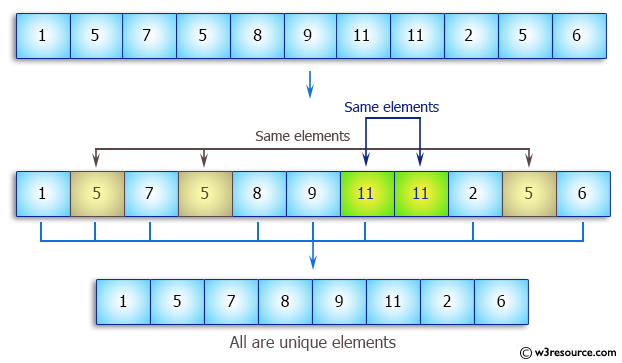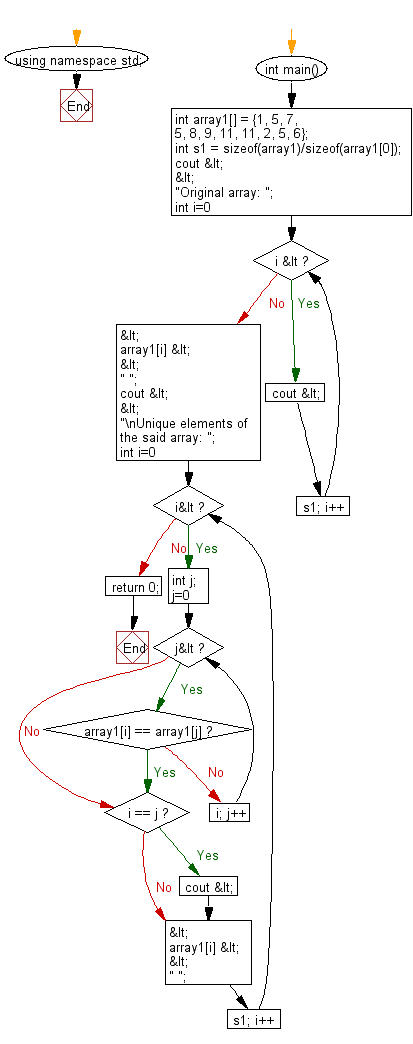﻿ C++ : Find and print all unique values of an array# C++ Exercises: Find and print all unique elements of a given array of integers

## C++ Array: Exercise-26 with Solution

Write a C++ program to find and print all unique elements of a given array of integers.

Pictorial Presentation:Sample Solution:

C++ Code :

``````#include <iostream>
using namespace std;

int main()
{
int array1[] = {1, 5, 7, 5, 8, 9, 11, 11, 2, 5, 6};
int s1 = sizeof(array1)/sizeof(array1);

cout << "Original array: ";

for (int i=0; i < s1; i++)
cout << array1[i] <<" ";

cout <<"\nUnique elements of the said array: ";
for (int i=0; i<s1; i++)
{
int j;
for (j=0; j<i; j++)
if (array1[i] == array1[j])
break;
if (i == j)
cout << array1[i] << " ";
}
return 0;
}
``````

Sample Output:

```Original array: 1 5 7 5 8 9 11 11 2 5 6
Unique elements of the said array: 1 5 7 8 9 11 2 6
```

Flowchart:C++ Code Editor:

What is the difficulty level of this exercise?

Test your Programming skills with w3resource's quiz.

﻿